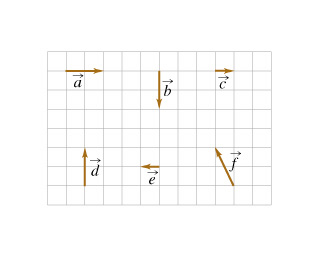# Problem: Six vectors (a through f ) have the magnitudes and directions indicated in the figure. (Figure 1)Rank the vector combinations on the basis of their magnitude. Rank from largest to smallest. To rank items as equivalent, overlap them.a+cf+cda+ba+ea+d

###### FREE Expert Solution

2D vector magnitude:

$\overline{)\mathbf{|}\stackrel{\mathbf{⇀}}{\mathbf{r}}\mathbf{|}{\mathbf{=}}\sqrt{{\mathbf{x}}^{\mathbf{2}}\mathbf{+}{\mathbf{y}}^{\mathbf{2}}}}$

a + c = 2i + 1i = 3i

|a + c| = 3

f + c = (-1i + 2j) + 1i = 2j

|f + c| = 2

d = 2j###### Problem Details

Six vectors (a through f ) have the magnitudes and directions indicated in the figure. (Figure 1)Rank the vector combinations on the basis of their magnitude.

Rank from largest to smallest. To rank items as equivalent, overlap them.

a+c

f+c

d

a+b

a+e

a+d